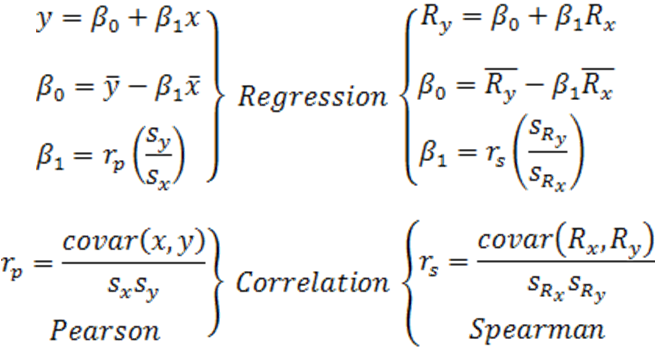We have updated and improved our Regression & Correlation Calculator to demonstrate, as shown in the above figure, that a Spearman’s Correlation Coefficient is just a Pearson’s Correlation Coefficient computed from ranks.

The tool uses an algorithm that converts values to ranks and averages any ties that might be present before calculating the correlations. This comes handy when we need to compute a Spearman’s Correlation Coefficient from ranks with a large number of ties.

We have explained in the “What is Computed?” section of the page’s tool that as the number of ties increases the classic textbook formula for computing Spearman’s correlationsincreasingly overestimates the results, even if ties were averaged.

By contrast, computing a Spearman’s as a Pearson’s always work, even in the presence or absence of ties.

To illustrate the above, consider the following two sets:

X = [1, 2, 3, 4, 5, 6, 7, 8, 9, 10]
Y = [1, 1, 1, 1, 1, 1, 1, 1, 1, 2]

using Spearman’s classic equation rs = 0.6364 ≈ 0.64.
By contrast, rs = 0.5222 ≈ 0.52 when computed as a Pearson coefficient derived from ranks. This is a non trivial difference.

Accordingly, we can make a case as to why we should ditch for good Spearman’s classic formula.

We also demonstrate in the page’s tool why we should never arithmetically add or average Spearman’s correlation coefficients. The same goes for Pearson’s.

Early articles in the literature of correlation coefficients theory failed to recognize the non-additivity of Pearson’s and Spearman’s Correlation Coefficients.

Sadly to say, this is sometimes reflected in current research articles, textbooks, and online publications. The worst offenders are some marketers and teachers that, in order to protect their failing models, resist to consider up-to-date research on the topic.

PS. Updated on 09-14-2018 to include the numerical example and to rewrite some lines.